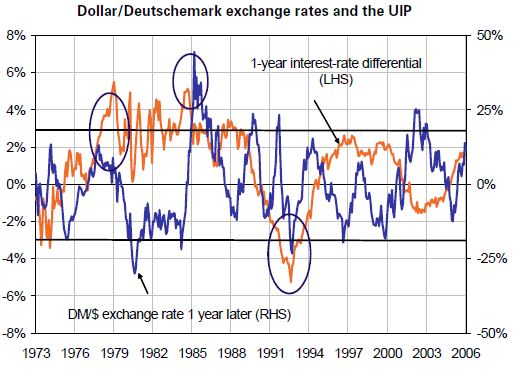The link between exchange rates and interest rates is a recurring topic of discussion among economists and financial analysts, with particular emphasis on how a monetary policy shift affects the exchange rate.

This article focus on the relationship referred to as uncovered interest-rate parity (UIP). UIP formalises the following principle: when two currencies' relative interest rates diverge substantially and durably, exchange rates will move so that a risk-free investment in one of the currencies will be equivalent to a risk-free investment of the same maturity in the other currency, otherwise it would become possible to achieve limitless returns at no risk.

Empirical studies show that this relationship between interest rates and exchange rates is better verified in the long run (beyond one year), and when interest-rate differentials are sufficiently large. In the short run, on the other hand, the relationship between interest rates and exchange rates in the developed countries is unstable and tends, on average, to display the opposite sign to the one predicted by the uncovered interest-rate parity relationship.

This short-term deviation may be due to the existence of shocks of a monetary origin, whose unexpected character may explain why the uncovered interest-rate parity is not verified ex post even though it remains an equilibrium relationship at each moment in time.

The introduction of agents' uncertainty as to future movements in financial variables, exchange rates in particular, in the presence of transaction costs, may also explain the phenomenon of deviation from the uncovered parity: agents' uncertainty would in that case prevent them from carrying out arbitrages arising from the deviation from the equilibrium relationship, thereby dampening speculation.